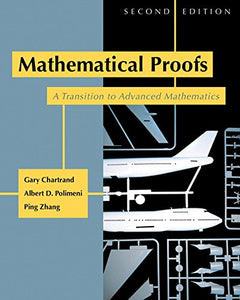Pearson

# Mathematical Proofs: A Transition To Advanced Mathematics (2Nd Edition)

• Publish Date: 2007-10-13
• Binding: Hardcover
• Author: Gary Chartrand;Albert D. Polimeni;Ping Zhang

Regular price \$17.71 Sale price \$202.21

Attention: For textbook, access codes and supplements are not guaranteed with used items.

Mathematical Proofs: A Transition to Advanced Mathematics,2/e, prepares students for the more abstract mathematics courses that follow calculus. This text introduces students to proof techniques and writing proofs of their own. As such, it is an introduction to the mathematics enterprise, providing solid introductions to relations, functions, and cardinalities of sets. KEY TOPICS: Communicating Mathematics, Sets, Logic, Direct Proof and Proof by Contrapositive, More on Direct Proof and Proof by Contrapositive, Existence and Proof by Contradiction, Mathematical Induction, Prove or Disprove, Equivalence Relations, Functions, Cardinalities of Sets, Proofs in Number Theory, Proofs in Calculus, Proofs in Group Theory. MARKET: For all readers interested in advanced mathematics and logic.